Courses

Lakhmir Singh & Manjit Kaur Test: Motion

20 Questions MCQ Test Science Class 9 | Lakhmir Singh & Manjit Kaur Test: Motion

Description
Attempt Lakhmir Singh & Manjit Kaur Test: Motion | 20 questions in 20 minutes | Mock test for Class 9 preparation | Free important questions MCQ to study Science Class 9 for Class 9 Exam | Download free PDF with solutions
QUESTION: 1

Solution:
QUESTION: 2

The slope of the x - t graph is a measure of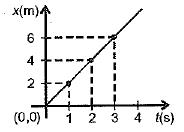Solution: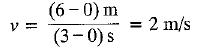QUESTION: 3

Odometer of automobiles records

Solution:
QUESTION: 4

Motion of bodies A and B is depicted by the x-t graph. Then which of the following is/are incorrect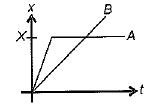A: A has uniform motion.
B: B has less velocity than A initially
C: B crosses A at X
D: A comes to rest at X

Solution:

According to the graph given, the body A does not have uniform motion. An object is said to be in uniform motion when it covers equal distances in equal intervals of time. In this case, it accelerates to a particular velocity and remains the same continuously. Otherwise, it is evident that the velocity of B is lesser that A initially and the body B crosses A at X.
Hence, the statement A has uniform motion is not correct.

QUESTION: 5

The speedometer of a car measures

Solution:
QUESTION: 6

​ From the x - t graph, one can draw the following conclusions ;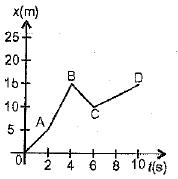A: vOA < vAB
B: vBC is negative
C: vCD is the least by magnitude
D: acceleration is uniform

Solution:
QUESTION: 7

If the displacement-time graph of a particle is parallel to the time axis, the velocity of the particle is

Solution:
QUESTION: 8

In a uniformly accelerated motion,
A: v - t graph is a straight line not parallel to t or v axis.
B: x - t graph is not a straight line.
C: Slope of v - t graph varies.
D: Slope of x - t graph is constant.

Solution:
QUESTION: 9

The velocity time graph of a moving particle is shown in Figure, The acceleration of the particle is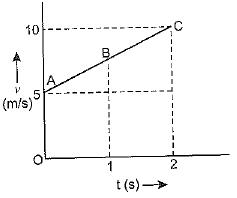Solution:
QUESTION: 10

If the velocity of a body is reducing, it is said to have

Solution:
QUESTION: 11

The velocity time (v - t) graph of a moving particle is shown in Figure. The acceleration is maximum for segment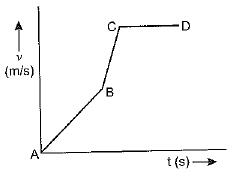Solution:
QUESTION: 12

One can conclude from the given x-t graph that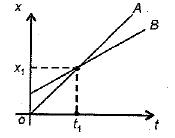Solution:
QUESTION: 13

The numerical ratio of displacement to distance for a moving object is

Solution:
QUESTION: 14

A body starting at a point, say A, reaches, say B, ahead in a straight line and returns back to A. Then there is

Solution:
QUESTION: 15

From the given v - t graph, it can be inferred that the object is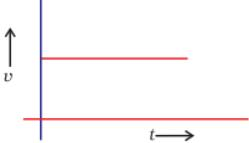Solution:

In the given graph we find that the velocity is constant throughout, hence the object represented in the v - t graph is said to be in uniform motion.  Zero acceleration is also called uniform acceleration.

QUESTION: 16

A body moves in a circle of radius 5 m with a speed of 5 ms-1. Then it has
A: an acceleration of 5 ms-2
B: an acceleration varying with direction alone

Solution:
QUESTION: 17

Four cars A, B, C and D are moving on a levelled road. Their distance versus time graphs are shown in figure.
Choose the correct statement.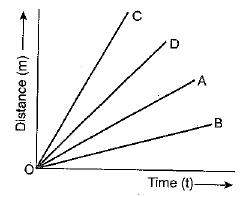Solution:
QUESTION: 18

The displacement of the body in 5 seconds from the beginning of the motion is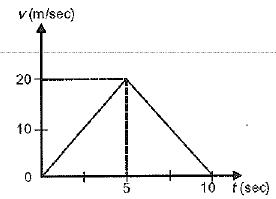Solution:

Displacement = Area of ΔABC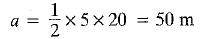QUESTION: 19

Suppose a boy is enjoying a ride on a merry go round which is moving with a constant speed of 10 ms-1. It implies that the boy is

Solution:

Acceleration is the measure of how fast the velocity changes. Velocity is a vector quantity and a change in velocity is due to  change in magnitude or change in direction or both. Here,  the boy is moving with constant speed but velocity changes and hence is supposed to be in accelerated motion.

QUESTION: 20

The v-t graph shown here depicts the motion of A and B such that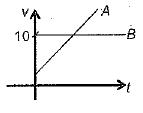Solution:Use Code STAYHOME200 and get INR 200 additional OFF Use Coupon Code

Track your progress, build streaks, highlight & save important lessons and more!

Similar Content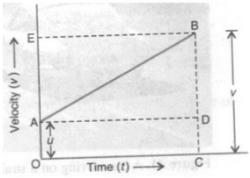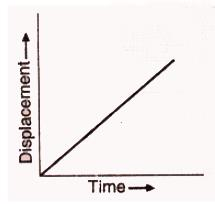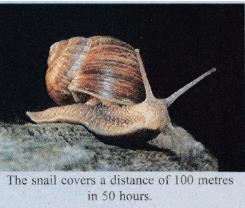Related tests